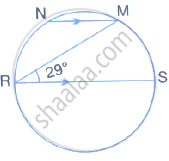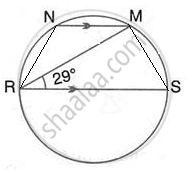Share

# In the Given Figure, Rs is a Diameter of the Circle. Nm is Parallel to Rs and ∠Mrs = 29°. Calculate: ∠Rnm, - Mathematics

Course

#### Question

In the given figure, RS is a diameter of the circle. NM is parallel to RS and ∠MRS = 29°.
Calculate:  ∠RNM,#### SolutionJoin RN and MS.

∴ ∠RMS = 90°

(Angle in a semicircle is a right angle)

∴ ∠RSM = 90° - 29° = 61°

(By angle sum property of triangle RMS)

∴ ∠RNM =180° ∠RSM =180° - 61° = 119°

(pair of opposite angles in a cyclic quadrilateral are supplementary)

Is there an error in this question or solution?